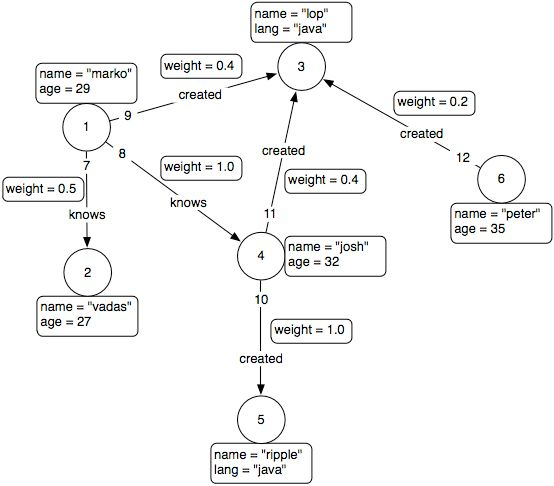Facilitating the Spread of Knowledge and Innovation in Professional Software Development

Write for InfoQ

### Topics

InfoQ Homepage News Gremlin, a Language for Working with Graphs

# Gremlin, a Language for Working with Graphs

This item in japanese

Gremlin is a Turing Complete programming language useful for working with graphs. It is a Java DSL that makes extensive use of XPath to query, analyze and manipulate graphs.

Gremlin can be used to create multi-relational graphs. Because the elements of the graph, vertices and edges, have properties defined as key-value pairs, the graph is called a property graph, and this is an example:The language has the following types:

• graph: a graph is composed of a set of vertices and a set of edges.
• vertex: a vertex is composed of a set of outgoing edges, incoming edges, and a map of properties.
• edge: an edge is composed of an outgoing vertex, incoming vertex, and a map of properties.
• boolean: a boolean can either be true or false.
• number: a number is a natural (integer) or real (double) number.
• string: a string is an array of characters.
• list: a list is an ordered collection of potentially duplicate objects.
• map: a map is an associative array from a set of object keys to a collection of object values.

Besides XPath’s mathematical operations – addition, subtraction, multiplication, etc. - , Gremlin has a number of statements like If/Else, While, Repeat, Foreach, and others.

Gremlin can be used against any framework that implements the General Graph Model. This model consists of a number of components - graph, element, vertex, edge, and index - and their associated Java interfaces which need to be implemented in order to manipulate a graph using Gremlin constructs.

One example of using Gremlin is working with graphs saved as MongoDB documents. Another is working with Resource Description Framework stores like OpenRDF, AllegroGraph, Open Virtuoso or the Neo4j graph database. In the future, the Gremlin team intends to add support for CouchDB and Terracotta.

Example

To work with a JSON encoding of a graph one needs to use the following schemas for vertices and edges:

object {   string "_id";   array { string } inEdges;   array { string } outEdges;   object { }* properties; };
object {   string "_id";   string label;   string inVertex;   string outVertex;   object { }* properties; };

Then, the graph pictured above is encoded in JSON as following:

//// VERTEX COLLECTION ////  {   _id: "1",    properties: {      name : "marko",      age : 29    },    outEdges : ["7","8","9"]  }  {   _id: "2",    properties: {      name : "vadas",      age : 27    },    inEdges : ["7"]    }   ... [section skipped for brevity]    //// EDGE COLLECTION ////  {   _id: "12",    label: "created",    properties: {      weight : 0.2    },    outVertex : "6",    inVertex : "3"  }

This data is entered into MongoDB which creates two collections, one for vertices and one for edges, and Gremlin allows the manipulation of these collections through the General Graph Model interface: adding/removing vertices or edges, getting a list of vertices or edges, getting/setting properties on vertices or edges, and navigating the graph by finding the associated edges to a vertex or the vertices of an edge.

Adding two vertices to a graph and connecting them through an edge named “related_to” is done as following:

 gremlin> $v := g:add-v($g) ==>v gremlin> $u := g:add-v($g) ==>v gremlin> $e := g:add-e($g, $v, 'related_to',$u) ==>e[0-related_to->1]

Graphs have many possible applications in computer chip design, biology, networks, etc. One simple example is creating a graph where vertices are represented by a website’s pages and edges exist between pages containing links to other pages. Gremlin allows to navigate and modify such a graph of pages and their properties.

Resources: TinkerGraph – a reference implementation of the General Graph Model, Gremlin Documentation, Gremlin User Group.

Style

## Hello stranger!

You need to Register an InfoQ account or or login to post comments. But there's so much more behind being registered.

Get the most out of the InfoQ experience.

Allowed html: a,b,br,blockquote,i,li,pre,u,ul,p